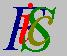Mauro Murzi's pages on Philosophy of Science - Quantum mechanics
Derivative of a complex functionComplex functions Partial derivatives# [2. Mathematical elements.]

## Derivative of a complex function.

The derivative of a function is the rate of change of the value of the function with respect to its argument. There is no substantial difference between the derivative of a real function and the derivative of a complex function, so it is possible to use the usual rules applicable to the derivative of a real function. There are some notations to denote the derivative of a function φ(x): the most common are φ'(x) , dφ(x) / dx , Dφ(x). Here is a small table with the derivative of some functions.

 Function Derivative Notes φ (x) = x n φ'(x) = n x n-1 n is a natural number φ (x) = sin(x) φ'(x) = cos(x) φ (x) = cos(x) φ'(x) = - sin(x) φ (x) = e x φ'(x) = e x e is the base of natural logarithms (e=2.718...) φ (x) = e - x φ'(x) = - e - x φ (x) = e k x φ'(x) = k e k x φ (x) = e i x φ'(x) = e i (x+π/2) φ (x) = e - i x φ'(x) = - e -i (2x+π) / 2 φ (x) = e k i x φ'(x) = k e i (kx+π/2) φ (x) = ln(x) φ'(x) = 1/x ln is the natural logarithm

Let φ (x) and Ψ (x) be two complex functions of a real variable. The derivatives of their addition, multiplication and division are:

D (φ (x) + Ψ (x)) =  φ'(x) + Ψ'(x)
D (φ (x) · Ψ (x)) =  φ (x) · Ψ'(x) +  φ'(x) · Ψ (x)
D (φ (x) / Ψ (x)) =  φ'(x) / Ψ (x) -  Ψ'(x) · φ (x) / (Ψ (x))²

The derivative φ'(x) of a function φ (x) is a function too; thus there exists also the derivative of φ'(x), which is called the second derivative of φ (x) and is usually denoted by one of the following expressions: φ"(x) , d²φ (x) / dx².Complex functions Partial derivatives